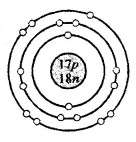# ICSE Selina Class 8 Chemistry Chapter 4- Atomic Structure

The Class 8 Chemistry Chapter 4- Atomic Structure, deal with the structure of atoms, electronic configuration, valency and more. First, study the chapter and learn the concepts thoroughly. Later, try answering the questions given in the Selina textbook. Once you are done with solving them, evaluate how right your answers are by comparing it with the ICSE Selina Class 8 Chemistry Chapter 4- Atomic Structure Solutions by BYJU’S.

Atomic number: The number of protons in an atom is known as the Atomic number (z).

Atomic number = Number of protons

Mass Number: The sum of the number of protons and neutrons present in the nucleus of an atom is known as the mass number (A).

Mass number = Protons + Neutrons

The chapter also deals with the how notation of element is done, and its electronic configuration, the arrangement of electrons in the shells.

Rules for accommodating electrons in various shells and the octet configuration are also explained clearly in the chapter. The chapter also describes, in details, the following concepts:

• Valence electrons: Valence electrons are the electrons present in the outermost shell of an atom.
• Valency: Valency is the combining capacity of the atoms to form molecules either with the same or different elements.

## Download ICSE Class 8 Chemistry Selina Solutions PDF for Chapter 4 – Atomic Structure

Question 1.

Fill in the blanks.

(a) Dalton said that atoms could not be divided

(b) An ion which has a positive charge is called a cation

(c) The outermost shell of an atom is known as valence shell

(d) The nucleus of an atom is very hard and dense.

(e) Neutrons are neutral particles having a mass equal to that of protons.

(f) Isotopes are the atoms of an element having the same atomic number but a different mass number.

Question 2.

Write ‘true’ or ‘false’ for the following statements:

(a) An atom, on the whole, has a positive charge.

False

(b) The maximum number of electrons in the first shell can be 8.

False

(c) The central pad of the atom is called the nucleus.

True.

Question 3.

Give the following a suitable word/phrase.

(a) The sub-atomic particle with a negative charge and negligible mass.

Solution: Neutron

(b) Protons and neutrons present in the nucleus.

Solution: Mass number

(c) The electrons present in the outermost shell.

Solution: Valency

(d) Arrangement of electrons in the shells of an atom.

Solution: Orbits or Valence shells

(e) The number of protons present in the nucleus of an atom.

Solution: Atomic number

(f) The sum of the number of protons and neutrons of an atom.

Solution: Mass number

(g) Atoms of the same element with same atomic number but a different mass number.

Solution: Isotopes

(h) The smallest unit of an element which takes part in a chemical reaction.

Solution: Atom

Question 4.

Multiple Choice Questions

(a) The outermost shell of an atom is known as

1. valency

2. valence electrons

3. nucleus

4. valence shell

(b) The number of valence electrons present in magnesium is

1. two

2. three

3. four

4. five

(c) The subatomic particle with a negative charge is

1. proton

2. neutron

3. electron

4. nucleon

(d) If the atomic number of an atom is 17 and the mass number is 35 then the number of neutrons will be

1. 35

2. 17

3. 18

4. 52

(e) The number of electrons in an atom is equal to the number of

1. protons in a neutral atom

2. neutrons in a neutral atom

3. nucleons in a neutral atom

4. none of the above

Answer: 1. protons in a neutral atom

(f) The sum of the number of protons and the number of neutrons present in the nucleus of an atom is called its

1. mass number

2. atomic number

3. number of electrons

4. all of the above

Question 5.

Name three fundamental particles of the atom. Give the symbol with charge, on each particle.

Solution:

The fundamental particles of the atom are electrons, protons and neutrons.

 Particle Symbol Charge Electron e_ -1 or -1.602×10-19 c. where-1 represent its one unit negative electrical charge Proton p+ +1 or 1.602×10-19 c.where1 represents one unite +ve electrical charge. Neutron n0 0

Question 6.

Define the following terms:

(a) Atomic number

(b) Mass number

(c) Nucleons

(d) Valence shell

Solution:

(a) Atomic number: The number of protons present in an atom is known as Atomic number. It is denoted by Z. Example: An atom of oxygen contains 8 protons. Therefore its atomic number is 8.

(b) Mass number: The sum of the number of protons and neutrons present in the nucleus of an atom is known as the Mass number and it is denoted by A.

I.e. Mass number = Number of protons + Number of neutrons.

(c) Nucleons: The protons and neutrons collectively are known as nucleons.

(d) Valence Shell: The outermost shell of an atom is known as its valence shell.

Question 7.

Mention briefly the salient features of Dalton’s atomic theory (five points).

Solution:

Salient features of Dalton’s atomic theory:

1. The matter has very small and indivisible particles called atoms, it can neither be created nor be destroyed.

2. The atoms of an element are alike in all aspects i.e. size, mass, density, chemical properties but they differ from the atoms of other elements.

3. Atoms of an element combine in small numbers to form molecules of the element.

4. Atoms of one element combine with atoms of another element in a simple number ratio to form molecules of compounds.

5. Atoms are the smallest units of matter that can take part in a chemical reaction during which the only rearrangement of atoms takes place.

Question 8.

(a) What are the two main features of Rutherford’s atomic model?

(b) State its one drawback.

Solution:

(a) According to Rutherford’s model, an atom consists of

• The centrally located nucleus
• The outer circular orbits

A positively charged mass is located centrally called nucleus. The entire mass of the atom is concentrated in it. It is the densest part of the atom. Its size is very small as compared to the atom as a whole.

Electrons revolve in circular orbits in the space available around the nucleus. An atom is electrically neutral i.e., the number of protons and electrons present in an atom are equal.

(b) The stability of the atom could not be explained by Rutherford’s atomic model as it is like a solar system, the sun is at the centre and the planets revolve around it, in an atom the electrons revolve around the centrally located nucleus containing protons.

Question 9.

What are the observations of the experiment done by Rutherford in order to determine the structure of an atom?

Solution:

Following were the observations made by Rutherford:

• Most of the alpha particle passed straight through the foil without any deflection from their path.
• Only a small fraction of alpha particles were deflected from their actual path by small angles.
• Only a few particles bounced back.

Question 10.

State the mass number, the atomic number of neutrons and electronic configuration of the following atoms.

$$\begin{array}{l}_{6}^{12}\textrm{C}\end{array}$$
$$\begin{array}{l}_{8}^{16}\textrm{O}\end{array}$$
$$\begin{array}{l}_{9}^{19}\textrm{F}\end{array}$$
$$\begin{array}{l}_{10}^{20}\textrm{Ne}\end{array}$$
$$\begin{array}{l}_{13}Al^{27}\end{array}$$
$$\begin{array}{l}_{17}Cl^{35}\end{array}$$

Also, draw atomic diagrams for them.

Solution:

 Name of elements Atomic number Atomic mass No. of proton No. of electrons No. of neutrons Electronic configuration $$\begin{array}{l}_{6}^{12}\textrm{C}\end{array}$$ 6 12 6 6 6 2,4 $$\begin{array}{l}_{8}^{16}\textrm{O}\end{array}$$ 8 16 8 8 8 2,6 $$\begin{array}{l}_{9}^{19}\textrm{F}\end{array}$$ 9 19 9 9 10 2,7 $$\begin{array}{l}_{10}^{20}\textrm{Ne}\end{array}$$ 10 20 10 10 10 2,8 $$\begin{array}{l}_{13}Al^{27}\end{array}$$ 13 27 13 13 14 2,8,3 $$\begin{array}{l}_{17}Cl^{35}\end{array}$$ 17 35 17 17 18 2,8,7

Carbon –

$$\begin{array}{l}_{6}^{12}\textrm{C}\end{array}$$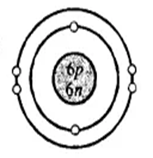Oxygen –

$$\begin{array}{l}_{8}^{16}\textrm{O}\end{array}$$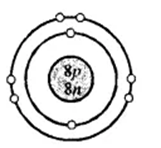Fluorine –

$$\begin{array}{l}_{9}^{19}\textrm{F}\end{array}$$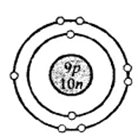Neon –

$$\begin{array}{l}_{10}^{20}\textrm{Ne}\end{array}$$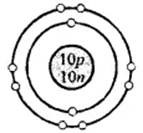Aluminium –

$$\begin{array}{l}_{13}Al^{27}\end{array}$$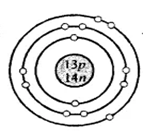Chlorine –

$$\begin{array}{l}_{17}Cl^{35}\end{array}$$